### Home > PC > Chapter 8 > Lesson 8.2.2 > Problem8-87

8-87.
1. Sketch the graphs of the following functions. Homework Help ✎

1. f(x) = −ex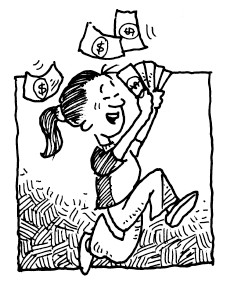2. g(x) = ex + 2

3. h(x) = ex+2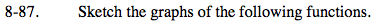Use the parent graph as a guide for transforming the graphs appropriately.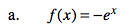The − sign reflects the graph across the x-axis.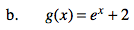The graph is shifted up 2 units.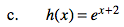The graph is shifted to the left 2 units.